e-ISSN: 2319-9849

All submissions of the EM system will be redirected to Online Manuscript Submission System. Authors are requested to submit articles directly to Online Manuscript Submission System of respective journal.

# Solvation of Oxytetracycline Hydrochloride in Ethanol-Water Mixed Solvents.

Esam A Gomaa1*, Elsayed M Abou Elleef2 and Elsayed T Helmy3

Chemistry Department, Faculty of Science, Mansoura University, 35516-Mansoura, Egypt.

Basic Science Department, Delta Higher Institute for Engineering & Technology, 35681- Dakhlia, Mansoura, Egypt

Mansoura College international language schools(MCILS) (American –IGCSE-British), Dakhlia ,Mansoura Egypt,15-Talkha

*Corresponding Author:
Esam A Gomaa
Chemistry Department
Faculty of Science
Mansoura University
35516-Mansoura, Egypt

Received: 06 June 2014 Accepted: 04 March 2014 Published: 09 March 2014

Visit for more related articles at Research & Reviews: Journal of Chemistry

## Abstract

The aim of this study was to determine the thermodynamic functions, Gibbs energy, enthalpy and entropy for the solution processes of oxytetracycline hydrochloride in the mixed solvent (ethanol+water) from solubility values obtained at temperatures ranging from 293.15 K to 308.15 K. The respective thermodynamic functions for mixing and solvation processes as well as the activity coefficients for the solute were calculated. The experimental results enable us to estimate the value of thermodynamic solubility product, Ksp(th), of oxytetracycline hydrochloride in the mixed solvent. In addition, Gibbs energy, enthalpy, entropy for the solution processes and free energies of transfer of oxytetracycline hydrochloride from water to ethanol solutions.

#### Keywords

Solubility, thermodynamic, solubility product; mixed solvent, oxytetracycline hydrochloride.

#### Introduction

The solubility of solutes of all kinds in mixed solvents is of great practical importance, since many industrial process as well as laboratory procedures . Most physicochemical properties of ionic solutions are influenced by ionic strength and the dielectric constant of the solvent. Indeed, in the context of solution chemistry, solvent polarity, the dielectric constant of the solvent, and the ionic strength of the medium are of great interest as a measure of the solvent ability to stabilize charges or dipoles. As an example, the dissolution of an ionic salt in a solvent or in a mixed solvent depends on the relative permittivity and the polarity of the solvent as well as on the lattice energy of the solute and the ionic strength of the medium [2,3,4].

The solvation of dissolved ions determines many of the properties of electrolyte solutions [5,6,7], including their redox, complexation, and kinetic behavior. Its particular importance to solubilities, the central topic of the International Symposium on Solubility Phenomena, is illustrated by the thermodynamic cycle in Fig. 1. As shown, the (standard) Gibbs energy of solution, ΔsolnG°, is seen to be the sum of (the negative of the) lattice energy of the salt, ΔlattG°, and the solvation energies of the ions, ΔsolvG°. This cycle makes it clear that the only reason that salts dissolve in solvents is because the very large lattice energies that are lost upon dissolution are compensated by the even larger solvation energies of the ions.

Figure 1: Plots of log Ksp. for oxytetracycline hydrochloride versus 1/T at different vol % of EtOHBecause both ΔlattG° and ΔsolvG° are very large (negative) quantities, typically of the order of –1000 kJ/mol, it follows that relatively small changes in either, for example, arising from minor changes in the nature of the solvent or the salt, can result in dramatic changes in solubility. Given thats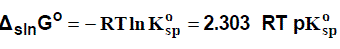where R and T have their usual meanings and Kosp is the (standard) solubility product of the salt:

In turn, most of the theories that have been used to predict the extend of solubility of an ionic compound in a given solvent or in a mixed solvent and related ion association are based on changes in the electrostatic properties of the solvent, solute and ion solvation as well as on the ionic strength of the medium [8,9,10,11,12,13,14,15].

#### Chemicals and Reagents

Oxytetracycline hydrochloride and ethanol were purchased from Merck Company with high degree of purity and used without further purification.

#### Preparation of mixed solvent and saturated solutions

The mixed solvent, (ethanol + water , 0, 20, 40, 60, 80 and 100% by volume), were prepared from bidistilled water and fairly pure ethanol. Then, saturated solutions of oxytetracycline hydrochloride were prepared by dissolving different amounts in closed test tubes containing different (ethanol + water) mixtures. These mixtures were then saturated with nitrogen gas as an inert atmosphere. The tubes were placed in a shaking thermostat (Model Gel) for a period of four days till equilibrium reached. The solubility, S (mole/Kg.solvent), of barium oxytetracycline hydrochloride in the mixed solvent at different temperature was determined gravimetrically by the solvent evaporating method. All the solubility experiments were repeated at least three times and the average were taken.

#### Result and Discussion

For an ionic compound, with the formula AB, we may consider the following equilibrium in its saturated solution at a given constant temperature.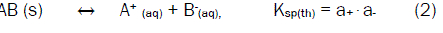Where Ksp(th) denotes the thermodynamic solubility product constant and a+ and a- refer to activity of A+ and B- in the solution, respectively. If the solubility of AB is very low, it may replace the activity of each ion by its concentration, so,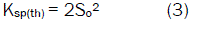Where So represents the molarity of BA in the very dilute solution. The electrostatic interaction becomes very small and the ion association phenomenon may be negligible (the activity coefficient γ±~1) [16,17,18,19,20,21].

At low concentration, the activity coefficient may be determined by using the Debye-Hückel limiting law: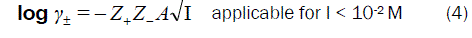Where Z+ and Z- are the charges of ions in solutions, A= 1.823×106(ε.T) -3/2 , the ionic strength, I, defined as, (Zi is the charge on ion i, and Where ,mi is the molality of ion i) and the ionic strength, I emphasizes the

charges of ions because the charge numbers occur as their squares. The values of dielectric constant (ε) for ethanol-water mixtures were taken from previous publications .

On the other hand, it can be considered that at high concentration, the solubility product constant (Ksp) is given by: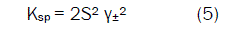where S is the molal solubility of AB and γ± is the mean molal activity coefficient. There are several theoreticallybased expressions that can be used to estimate single ion activity coefficients . At relatively high concentration, the electrostatic interaction becomes very large [23,24,25,26]. The activity coefficient may be determined using the extended Debye-Hückel law:Where B = 50.29 (ε.T)-½, and rε is the solvated radius.

At high concentrations, activity coefficients of electrolyte solutions can be determined by using the Davies equation  which is an empirical extension of Debye–Hückel theory. The final form of the equation gives the mean molal activity coefficient, γ±, of an electrolyte which dissociates into ions having charges Z+ and Z- as a function of ionic strength, I.The second term, 0.3 I, goes to zero as the ionic strength equal to zero, so the equation reduces to the Debye–Hückel equation at low concentration. However, as concentration increases, the second term becomes increasingly important, so the Davies equation can be used for solutions too concentrated to allow the use of the Debye–Hückel equation.

The molal solubility, S (mole/Kg.solvent), of oxytetracycline hydrochloride in the mixed solvent at different temperature was determined gravimetrically by the solvent evaporating method. The solubility values and activity coefficients are cited in Table (1).

Table 1: Solubility and molal activity coefficients for oxytetracycline hydrochloride in mixed EtOH-H2O solvents at different temperature.

The solubility of saturated solution of oxytetracycline hydrochloride in mixed solvents found to decrease with the increase the mole fraction of EtOH in the mixed solvents and increase with increase temperature depends primarily on the solvation of the solutes or their constituent ions by the components of the solvent mixtures. The saturated solution of oxytetracycline hydrochloride salt in the mixed solvent is perfectly non-ideal. The non-ideality is partly due to the mean activity coefficient of ions in the solution and partly due to the ion association phenomenon. The solubility product was calculated by the use of equation (8)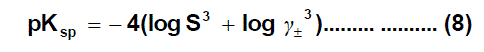solubility product (pKsp) data are given in Table (2), the Gibbs free energies of solvation and the transfer Gibbs free energies from water to mixed solvents were calculated by using equations (8) and (9) [28,29,20,31,32]. Their values are tabulated also in Table (2) and Table (3).

Table 2: Solubility product and Gibbs free energies of solvation for oxytetracycline hydrochloride in mixed EtOH-H2O solvents at different temperature.

Table 3: Transfer Gibbs free energies, enthalpy and entropy of solvation for oxytetracycline hydrochloride in mixed EtOH-H2O solvents at different temperature.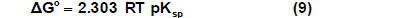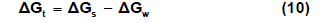Where (s), (w) denote solvent and water, respectively.

The enthalpies change of solvation (ΔH)s were calculated from the plots of log Ksp. versus 1/T as shown in Figs. 1, where the slope equals -ΔH/2.303 R using van 't Hoff equation :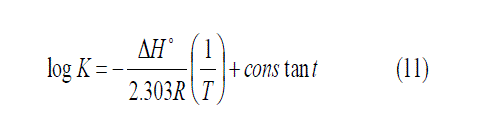From equation (10) , the values of (ΔG)s and (ΔH)s can be used to calculate the values of TΔS at different temperatures according to Gibbs-Helmholtz equation (12)  and all the results are reported in Table (3).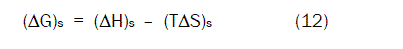The magnitude of ΔGt (solute, w → w + s) as shown in Figs. 2 is a measure of the overall change in the solvation (energy) of an ion upon its transfer from water to an organic–aqueous mixture. Such quantities are usually “well behaved” in the sense that they vary smoothly as a function of solvent composition, even though they may show great diversity.

Figure 2: Gibbs free energies of transfer (ΔGt) for oxytetracycline hydrochloride versus the mole fraction (Xs) of EtOH at different temperature.

It was concluded that the Gibbs free energies of transfer increase in negativity by increasing the mole fraction of EtOH in the mixed EtOH -H2O solvents indicating the decrease in spontaneous nature of oxytetracycline hydrochloride solubilization. This is due to more solvation behaviour in water than that of the mixed solvents where the Gibbs free energy values provide information on whether the process conditions favor or disfavor oxytetracycline hydrochloride solubilization in the aqueous carrier solution. Positive Gibbs free energy values indicate disfavourable conditions.

#### Conclusions

The solubility of saturated solution of oxytetracycline hydrochloride in mixed solvents found to decrease with the increase the mole fraction of EtOH in the mixed solvent and increase with increasing the temperature. In addition, Gibbs energy, enthalpy, entropy for the solution processes and free energies of transfer were also calculated in order to estimate the contribution of solute-solvent interactions related ion association are based on changes in the electrostatic properties of the solvent, solute and ion solvation as well as on the ionic strength of the medium. The saturated solution of oxytetracycline hydrochloride salt in the mixed solvent is perfectly non-ideal. The non-ideality is partly due to the mean activity coefficient of ions in the solution and partly due to the ion association phenomenon. By choosing a suitable model for estimating the mean activity coefficient and using the iteration calculations, we obtained the value of ion-pair concentration and the activity coefficient contribution and ion association contribution to the solubility of the considered ionic compound in the considered mixed solvent at different temperature.

#### Acknowledgements

This work was supported by Chemistry Department, Faculty of Science, Mansoura University and Basic Science Department, Delta Higher Institute for Engineering and Technology, Talkha, Mansoura, Egypt.

Replica watches